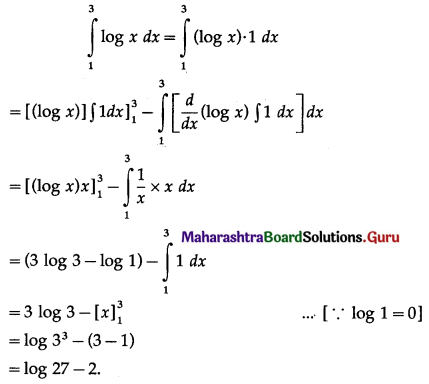# Maharashtra Board 12th Commerce Maths Solutions Chapter 6 Definite Integration Ex 6.1

Balbharati Maharashtra State Board Std 12 Commerce Statistics Part 1 Digest Pdf Chapter 6 Definite Integration Ex 6.1 Questions and Answers.

## Maharashtra State Board 12th Commerce Maths Solutions Chapter 6 Definite Integration Ex 6.1

Evaluate the following definite integrals:

Question 1.
$$\int_{4}^{9} \frac{1}{\sqrt{x}} d x$$
Solution: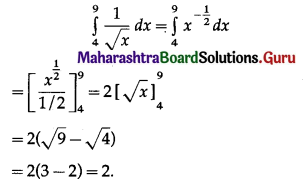Question 2.
$$\int_{-2}^{3} \frac{1}{x+5} d x$$
Solution: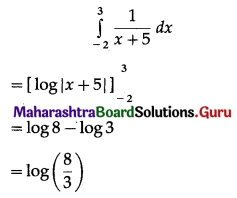Question 3.
$$\int_{2}^{3} \frac{x}{x^{2}-1} d x$$
Solution: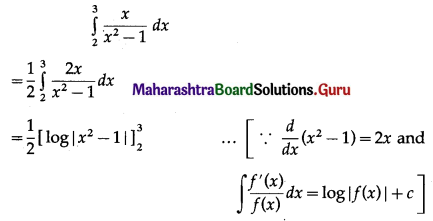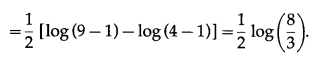Question 4.
$$\int_{0}^{1} \frac{x^{2}+3 x+2}{\sqrt{x}} d x$$
Solution: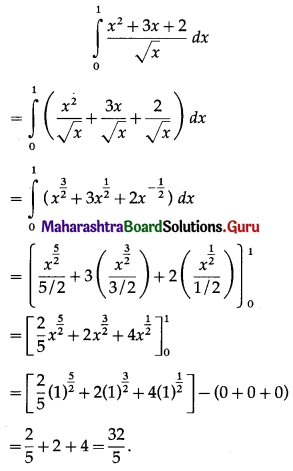Question 5.
$$\int_{2}^{3} \frac{x}{(x+2)(x+3)} d x$$
Solution:
Let I = $$\int_{2}^{3} \frac{x}{(x+2)(x+3)} d x$$
Let $$\frac{x}{(x+2)(x+3)}=\frac{A}{x+3}+\frac{B}{x+2}$$
∴ x = A(x + 2) + B(x + 3)
Put x + 3 = 0, i.e. x = -3, we get
-3 = A(-1) + B(0)
∴ A = 3
Put x + 2 = 0, i.e. x = -2, we get
-2 = A(0) + B(1)
∴ B = -2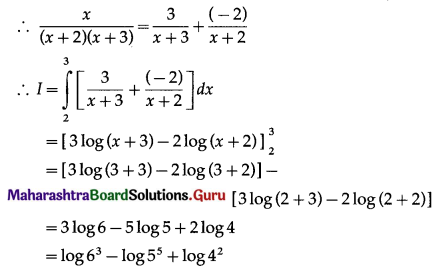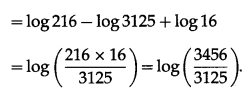Question 6.
$$\int_{1}^{2} \frac{d x}{x^{2}+6 x+5}$$
Solution: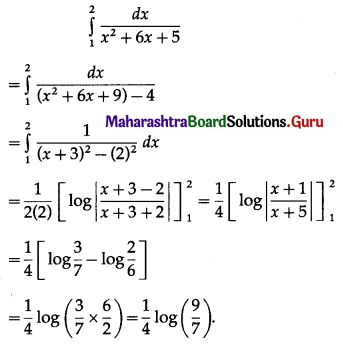Question 7.
If $$\int_{0}^{a}(2 x+1) d x$$ = 2, find the real values of ‘a’.
Solution:
Let I = $$\int_{0}^{a}(2 x+1) d x$$
= $$\left[2 \cdot \frac{x^{2}}{2}+x\right]_{0}^{a}$$
= a2 + a – 0
= a2 + a
∴ I = 2 gives a2 + a = 2
∴ a2 + a – 2 = 0
∴ (a + 2)(a – 1) = 0 1
∴ a + 2 = 0 or a – 1 = 0
∴ a = -2 or a = 1.

Question 8.
If $$\int_{1}^{a}\left(3 x^{2}+2 x+1\right) d x$$ = 11, find ‘a’.
Solution:
Let I = $$\int_{1}^{a}\left(3 x^{2}+2 x+1\right) d x$$
= $$\left[3\left(\frac{x^{3}}{3}\right)+2\left(\frac{x^{2}}{2}\right)+x\right]_{1}^{a}$$
= $$\left[x^{3}+x^{2}+x\right]_{1}^{a}$$
= (a3 + a2 + a) – (1 + 1 + 1)
= a3 + a2 + a – 3
∴ I = 11 gives a3 + a2 + a – 3 = 11
∴ a3 + a2 + a – 14 = 0
∴ (a3 – 8) + (a2 + a – 6) = 0
∴ (a – 2)(a2 + 2a + 4) + (a + 3)(a – 2) = 0
∴ (a – 2)(a2 + 2a + 4 + a + 3) = 0
∴ (a – 2)(a2 + 3a + 7) = 0
∴ a – 2 = 0 or a2 + 3a + 7 = 0
∴ a = 2 or a = $$\frac{-3 \pm \sqrt{9-28}}{2}$$
The latter two roots are not real.
∴ they are rejected.
∴ a = 2.Question 9.
$$\int_{0}^{1} \frac{1}{\sqrt{1+x}+\sqrt{x}} d x$$
Solution: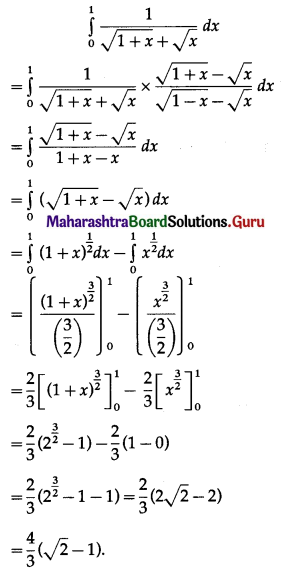Question 10.
$$\int_{1}^{2} \frac{3 x}{9 x^{2}-1} d x$$
Solution:
Let I = $$\int_{1}^{2} \frac{3 x}{9 x^{2}-1} d x$$ = $$\int_{1}^{2} \frac{3 x}{(3 x)^{2}-1} d x$$
Put 3x = t
∴ 3 dx = dt
∴ dx = $$\frac{d t}{3}$$
When x = 1, t = 3 × 1 = 3
When x = 2, t = 3 × 2 = 6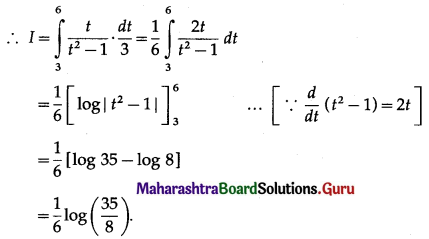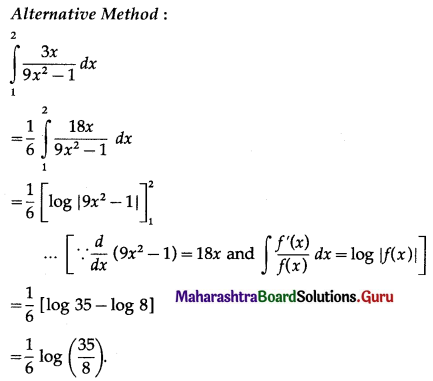Question 11.
$$\int_{1}^{3} \log x d x$$
Solution: